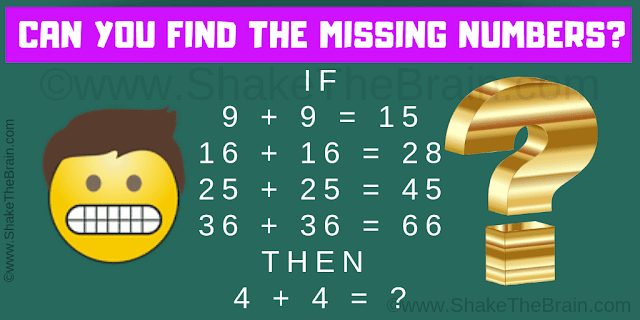## Tuesday, October 29, 2019

Time to put your logical reasoning to test. Here is the picture puzzle which will test your logical reasoning. In this picture puzzle, there are some logical number equations. These equations are incorrect Mathematically but these equations are correct logically. Try to find the logic of these equations and then solve the last equation.Can you solve this Mind Game Math Puzzle?
1.2.